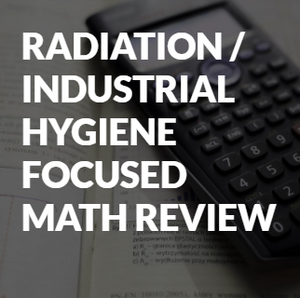# Radiation/Industrial Hygiene Focused Math Review

Regular price
\$75.00
Sale price
\$75.00

This course will cover math topics that are fundamental to performing calculations in many disciplines, including health physics and industrial hygiene.

Math topics that will be covered within this course:

• Significant Figures
• Order of Operation
• Fundamentals
• Fractions
• Decimals
• Percent
• Signed Numbers
• Geometry
• Trigonometry
• Scientific Fundamentals
• Scientific Notation
• Exponentials
• Logarithms
• Exponential Equations
• Dimensional Analysis
• Linear Interpolation